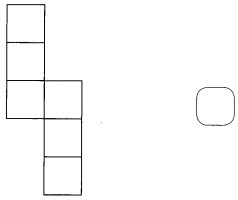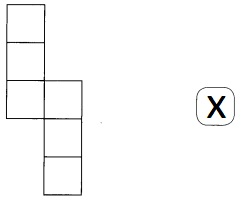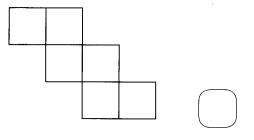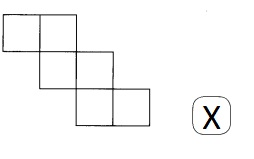# Math in Focus Grade 5 Chapter 14 Answer Key Three-Dimensional Shapes

This handy Math in Focus Grade 5 Workbook Answer Key Chapter 14 Three-Dimensional Shapes provides detailed solutions for the textbook questions.

## Math in Focus Grade 5 Chapter 14 Answer Key Three-Dimensional Shapes

Challenging Practice

Find the number of cubes in each prism.

Question 1.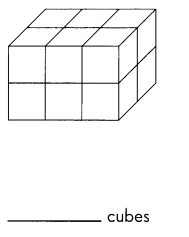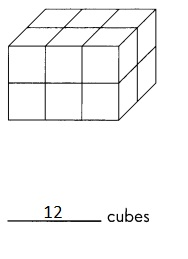Explanation:
Given solid cube has 3 cms X 2 cms X 2 cms each.
So the volume of given cube is  (3 cms X 2 cms X 2 cms)  =
12 cubes with 1 cm X 1 cm X 1 cm each.

Question 2.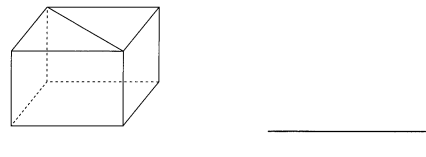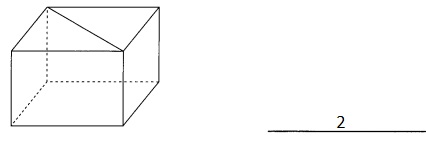Explanation:
Given cube is divided in center so we get 2 triangular prisms.

Each solid is cut vertically along the line shown. Identify the solid shapes that result.

Question 3.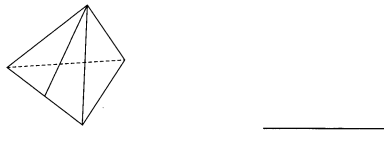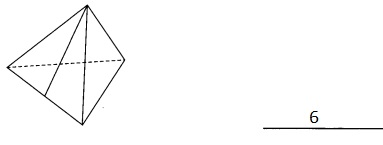Explanation:
Given solid shape has 3 triangular prisms again 3 have been divided from center
giving 6 solid shape prisms.
Question 4.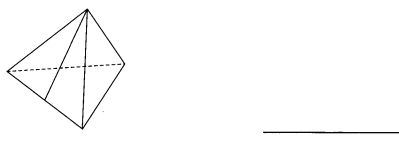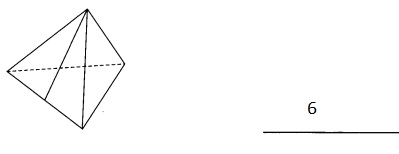Explanation:
Given solid shape has 3 irregular triangular prisms again 3 prisms have been
divided from center giving 6 solid shape small prisms.

Problem Solving

Which of these are nets of a cube? Check the boxes.

Question 1.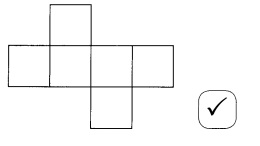Explanation:
Given nets form a cube so checked and ticked in the checkbox.

Question 2.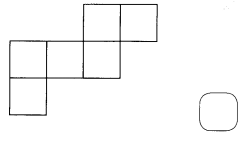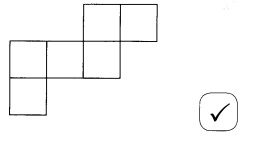Explanation:
Given nets form a cube so checked and ticked in the checkbox.

Question 3.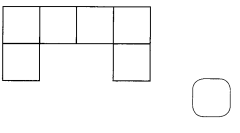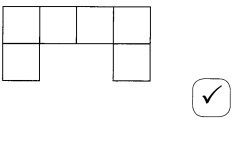Explanation:
Given nets form a cube so checked and ticked in the checkbox.

Question 4.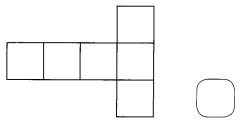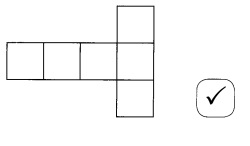Explanation:
Given nets form a cube so checked and ticked in the checkbox.

Question 5.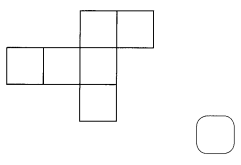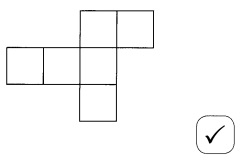Explanation:
Given nets form a cube so checked and ticked in the checkbox.

Question 6.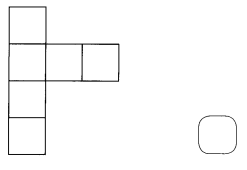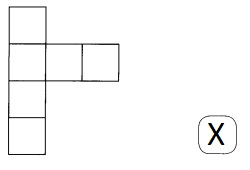Explanation:
Given nets cannot form a cube so checked and ticked cross in the checkbox.

Question 7.